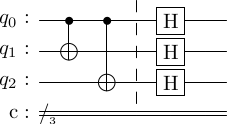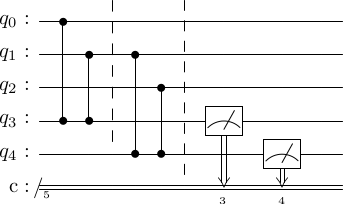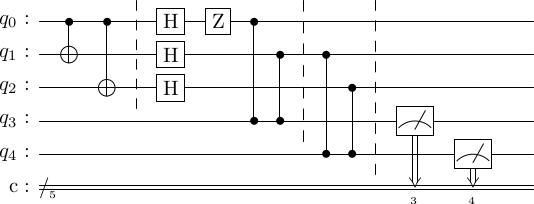# Quantum repetition code for phase-flips#

The code presented in the previous notebook could only correct for $X$ errors. Now, we present a code that can only correct for $Z$ errors. In the next notebook, we will combine these codes together to correct for both $X$ and $Z$ errors and more.

Alice, again wants to send a one-qubit state $\ket{\psi}$ to Bob, but via a channel that applies the $Z$ operator to qubits with probability $p$. This results in the phase flip, \begin{equation} \ket{\tilde\psi} = Z\ket{\psi} = Z(\alpha\ket{0} + \beta\ket{1}) = \alpha\ket{0} - \beta\ket{1}. \end{equation} As before, Bob has no way of knowing if any errors occured.

### Encoding#

To protect against such type of errors, we will employ a repetition code, but with a different basis. Now, \begin{align} \ket{0} \to \ket{\bar{0}} = \ket{+++}, \\ \ket{1} \to \ket{\bar{1}} = \ket{---}. \end{align} For this code basis, a qubit in state $\ket{\psi}$ is encoded as \begin{equation} \ket{\psi}\ket{00} = (\alpha\ket{0} + \beta\ket{1})\ket{00} \to \ket{\bar\psi} = \alpha\ket{+++} + \beta\ket{---}. \end{equation} This is done via the following circuit.

import stac
enc_circ = stac.Circuit()
enc_circ.append('CX', 0, 1)
enc_circ.append('CX', 0, 2)
enc_circ.append('TICK')
for i in range(3):
enc_circ.append('H', i)
enc_circ.draw()Determine logical gate operations $\bar{X}$ and $\bar{Z}$ for the three-qubit repetition code for phase-flips. These are operations that act on the logical basis $\set{\ket{\bar{0}}, \ket{\bar{1}}}$, in the normal way, i.e. \begin{align} \bar{X}\ket{\bar{0}} &= \ket{\bar{1}}, \quad \bar{X}\ket{\bar{1}} = \ket{\bar{0}}, \\ \bar{Z}\ket{\bar{0}} &= \ket{\bar{0}}, \quad \bar{Z}\ket{\bar{1}} = -\ket{\bar{1}}. \end{align} They can be constructed by some combination of operations on the three physical qubits. You will discover that there are possibly multiple ways of doing so.

### Errors#

The possible errors on the encoded state are given as follows.

Error Probability
$I$ $(1-p)^3$
$Z_1$ $p(1-p)^2$
$Z_2$ $p(1-p)^2$
$Z_3$ $p(1-p)^2$
$Z_1Z_2$ $p^2(1-p)$
$Z_1Z_3$ $p^2(1-p)$
$Z_2Z_3$ $p^2(1-p)$
$Z_1Z_2Z_3$ $p^3$

Determine the impact of each error on the input basis states.

Let input state be $\ket{000}$

Error Output state
$I$ $\ket{+++}$
$Z_0$ $\ket{+++}$
$Z_1$ $?$
$Z_2$ $?$
$Z_0Z_1$ $?$
$Z_0Z_2$ $?$
$Z_1Z_2$ $?$
$Z_0Z_1Z_2$ $?$

Let input state be $\ket{111}$

Error Output state
$I$ $\ket{---}$
$Z_0$ $\ket{+--}$
$Z_1$ $?$
$Z_2$ $?$
$Z_0Z_1$ $?$
$Z_0Z_2$ $?$
$Z_1Z_2$ $?$
$Z_0Z_1Z_2$ $?$

Do different errors result in the same output state?

As before, we will assume that $p$ is small, so we will only attempt to correct the errors $\set{I,Z_1,Z_2,Z_3}$. The effect of the $Z$ errors is to flip the state between plus and minus. So, for instance, \begin{equation} Z_1\ket{\bar{\psi}} = \alpha Z_1\ket{+++} + \beta Z_1\ket{---} = \alpha\ket{-++} + \beta\ket{+--}. \end{equation}

### Decoding#

The error detection strategy is the same as before. Bob employs a circuit that compares the value of two pairs of qubits, but in the plus/minus basis. This is accomplished using the following circuit.

synd_circ = stac.Circuit()
synd_circ.append('CZ', 0, 3)
synd_circ.append('CZ', 1, 3)
synd_circ.append('TICK')
synd_circ.append('CZ', 1, 4)
synd_circ.append('CZ', 2, 4)
synd_circ.append('TICK')
synd_circ.append('M', 3)
synd_circ.append('M', 4)
synd_circ.draw()To determine the syndromes, we can do the following

circ = stac.Circuit()
# encode the 0 state
circ += enc_circ

circ.append('Z', 0)

# do a syndrome measurement
circ += synd_circ

# draw to make sure you we understand what is happending
circ.draw()

# sample the output 10 times
circ.sample(10)[0 0]
[0 0]
[0 0]
[0 0]
[0 0]
[0 0]
[0 0]
[0 0]
[0 0]
[0 0]


Fill the following table

Syndrome Inferred error
$00$ $I$
$01$
$10$
$11$

In this code, if Bob infers that errors $Z_i$ occurs, than he applies $Z_i$ to the corrupted codeword to fix it.

### Bit-flip errors#

We won't belabor the point that this code cannot fix bit-flip errors.